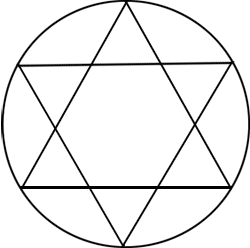## canvas 2D炫酷动效的实现套路和需要的技术积累

### 二、canvas动效眼见为实

Gif截屏效果如下：### 三、canvas 2D炫酷动效的实现的基本套路

#### 1. 了解canvas画布的工作原理

```var canvas = document.querySelector('canvas');
var context = canvas.getContext('2d');
// 清除画布
context.clearRect(0, 0, canvas.width, canvas.height);```

#### 2. canvas画布的不断绘制

```// requestAnimationFrame的向下兼容处理
if (!window.requestAnimationFrame) {
window.requestAnimationFrame = function(fn) {
setTimeout(fn, 17);
};
}```

```var canvas = document.querySelector('canvas');
var context = canvas.getContext('2d');

// 画布渲染
var render = function () {
// 清除画布
context.clearRect(0, 0, canvas.width, canvas.height);
// 绘制
draw();
// 继续渲染
requestAnimationFrame(render);
};
render();```

#### 4. 需要一个运动坐标变量

```var canvas = document.querySelector('canvas');
var context = canvas.getContext('2d');

// 坐标变量
var x = 0;
// 绘制方法
var draw = function () {
ball.x = x;
};
// 画布渲染
var render = function () {
// 清除画布
context.clearRect(0, 0, canvas.width, canvas.height);
// 位置变化
x++;
// 绘制
draw();
// 继续渲染
requestAnimationFrame(render);
};

render();```

#### 5. 实例对象管理canvas图形颗粒变量

```var Ball = function () {
this.x = 0;
this.draw = function () {
// 根据此时x位置重新绘制圆圈圈
// ...
};
};```

```var canvas = document.querySelector('canvas');
var context = canvas.getContext('2d');

// 存储实例
var store = {};
// 实例方法
var Ball = function () {
this.x = 0;
this.draw = function () {
// 根据此时x位置重新绘制圆圈圈
// ...
};
};
// 假设就一个圆圈圈
store = new Ball();
// 绘制画布上所有的圆圈圈的方法
var draw = function () {
// 位置变化
store.x++;
// 根据新位置绘制圆圈圈
store.draw();
};
// 画布渲染
var render = function () {
// 清除画布
context.clearRect(0, 0, canvas.width, canvas.height);
// 绘制画布上所有的圆圈圈
draw();
// 继续渲染
requestAnimationFrame(render);
};

render();```

#### 6. 随机多个实例对象坐标尺寸透明度等属性

```var canvas = document.querySelector('canvas');
var context = canvas.getContext('2d');

// 存储实例
var store = {};
// 实例方法
var Ball = function () {
// 随机x坐标也就是横坐标，以及变化量moveX，以及半径r
this.x = Math.random() * canvas.width;
this.moveX = Math.random();
this.r = 5 + 5 * Math.random();

this.draw = function () {
// 根据此时x位置重新绘制圆圈圈
// ...
};
};
// 假设10个圆圈圈
for (var indexBall = 0; indexBall < 10; indexBall += 1) {
store[indexBall] = new Ball();
}
// 绘制画布上10个圆圈圈
var draw = function () {
for (var index in store) {
// 位置变化
store[index].x += store[index].moveX;
if (store[index].x > canvas.width) {
// 移动到画布外部时候从左侧开始继续位移
store[index].x = -2 * store[index].r;
}
// 根据新位置绘制圆圈圈
store[index].draw();
}
};
// 画布渲染
var render = function () {
// 清除画布
context.clearRect(0, 0, canvas.width, canvas.height);
// 绘制画布上所有的圆圈圈
draw();
// 继续渲染
requestAnimationFrame(render);
};
render();```

#### 7. 补全圆圈圈的canvas绘制

`canvas`画圆直接API手册上就有，例如这里：

```var Ball = function () {
// 随机x坐标也就是横坐标，以及变化量moveX，以及半径r
this.x = Math.random() * canvas.width;
this.moveX = Math.random();
this.r = 5 + 5 * Math.random();

this.draw = function () {
// 根据此时x位置重新绘制圆圈圈
context.beginPath();
context.fillStyle="#369";
context.arc(this.x, canvas.height / 2, this.r, 0, Math.PI*2);
context.closePath();
context.fill();
};
};```

### 四、canvas 2D炫酷动效需要的技术积累

#### 4. 了解canvas所有API，尤其基本的绘图API

```// 开始路径绘制
context.beginPath();
// 虚线设定
context.setLineDash([2,2]);
// 设置路径起点
context.moveTo(x0, y0);
// 绘制一条到终点点的直线
context.lineTo(x1, y1);
// 设置线宽
context.lineWidth = 1.0;
// 设置线的颜色
context.strokeStyle = '#fff';
// 进行线的着色，这时整条线才变得可见
context.stroke();```

#### 5. 掌握常见的曲线函数```x=a+r*cosθ
y=b+r*sinθ```

```var arrPos = [
[a + r * Math.cos(0), b + r * Math.sin(0)],
[a + r * Math.cos(Math.PI * 2 / 3), b + r * Math.sin(Math.PI * 2 / 3)],
[a + r * Math.cos(Math.PI * 2 / -3), b + r * Math.sin(Math.PI * 2 / -3)],
[a + r * Math.cos(Math.PI / 3), b + r * Math.sin(Math.PI / 3)],
[a + r * Math.cos(Math.PI / -3), b + r * Math.sin(Math.PI / -3)],
[a + r * Math.cos(Math.PI), b + r * Math.sin(Math.PI)]
]```

```context.beginPath();
context.moveTo(arrPos, arrPos);
context.lineTo(arrPos, arrPos);
context.lineTo(arrPos, arrPos);
context.closePath();
context.fillStyle = '#fff';
context.fill();

context.beginPath();
context.moveTo(arrPos, arrPos);
context.lineTo(arrPos, arrPos);
context.lineTo(arrPos, arrPos);
context.closePath();
context.fillStyle = '#fff';
context.fill();```

### 五、接在结尾的结束语（本篇完）

﻿
1. 土豆土豆啊说道：

感谢旭哥的耐心分享 最近想认真仔细的学习一下canvas动画 和 canvas交互

2. nicholasurey说道：

赞~ 圆圈圈，角星星

3. miku说道：

大大 我想问的是 复杂度比较高的canvas对网站性能影响大不大？用flash和css3做的动效只要时间长了很明显会感觉到对网站性能的拖累

4. lc说道：

看来大神惜字如金啊

5. 星座变化说道：

张大大，请问你的每个星座中的每个星星的坐标是怎么定义的呢？

6. canvas动画resquestAnimationFrame()方法求教说道：

一直关注你的博客，最近在做一个canvas动画的小项目。对于resquestAnimationFrame（）函数运用感到不解，或者说是迷茫。查找资料发现比较少恳请博主有时间、有兴趣更新一些这方面的文章。

7. 喝茶的螃蟹说道：

张大大，请问怎么判断一个动画已经结束了呢？ 如您文章中的 星座A 变换到星座B 再变成星座C。怎么样才能判定从A到B的动画已经结束了？

• 张 鑫旭说道：

递增的变量值和你设定的最大数值一样的时候。

8. billzbc说道：

谢谢鑫哥深入浅出的讲解！受益非凡

9. 菜鸟说道：

学习了！谢谢你写的教程，很有用！

10. Tony说道：

数学不好，荒废了公式。。。。

11. 唐小狼说道：

我可以转载吗？

12. dont说道：

第三节-第六点里代码 random写错了 —> this.r = 5 + 5 * Math.randow();

第四节-第五点 “再六角星中” = =

13. 麦子说道：

大神，请问requestAnimFrame的速度如何设置，我想放慢点，可是找不到方法呀

14. 谢谢 学习了说道：

谢谢 学习了